# OECD Adult education level data analysis 6 - Time series regression analysis using RUnsplashJohannes Wが撮影した写真

This post is following of the above post.

In this post, I will do time series regression analysis.

Before starting this, let's see which LOCATION has the most obserbations.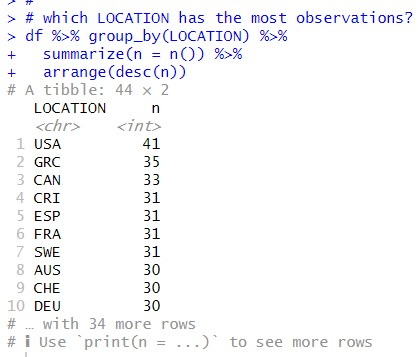USA has 41 observations. So I will use USA for time series regression analysis.

I make USA only data.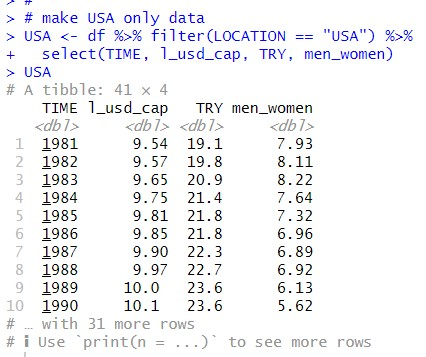Then, I make ts object from USA data frame.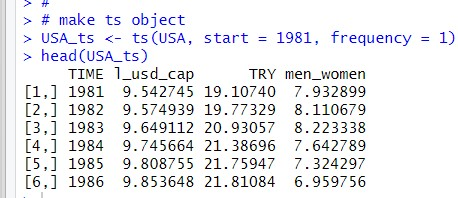Let's use plot() function with USA_ts object.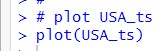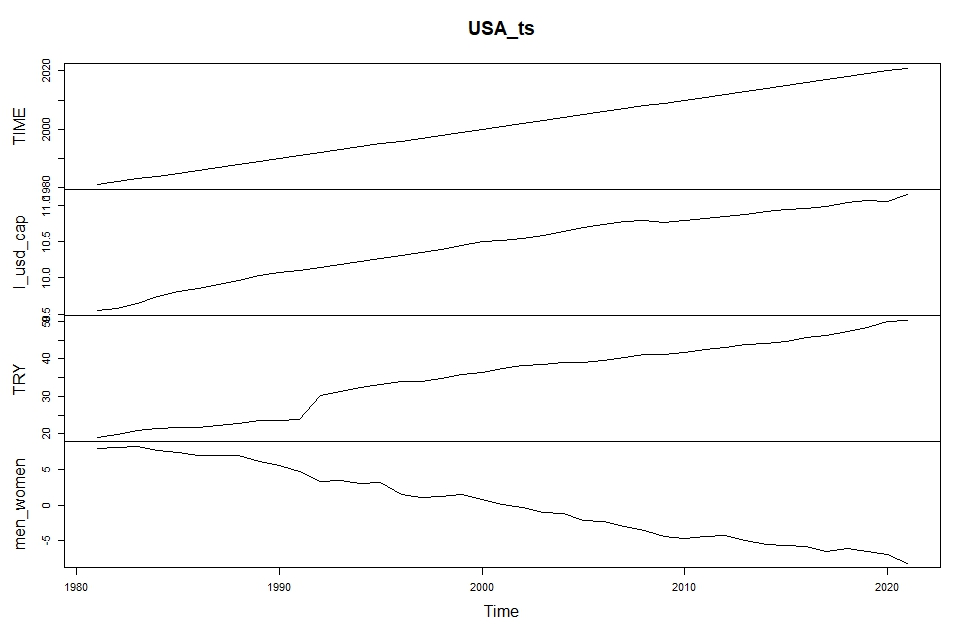l_usd_cap, TRY and men_women have almost linear trends.

So, I think it is better to use differenced data, X(t) - X(t-1).

I use diff() function to make differenced variables.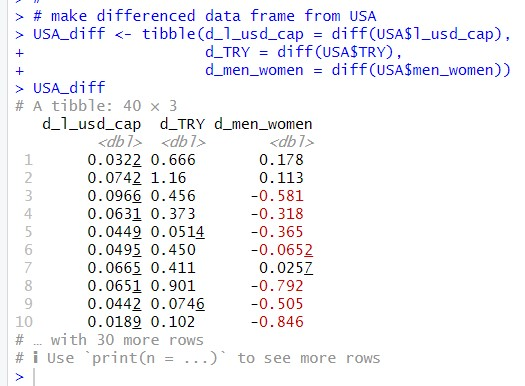Then, I make ts object from USA_diff. This time, start year should be 1982.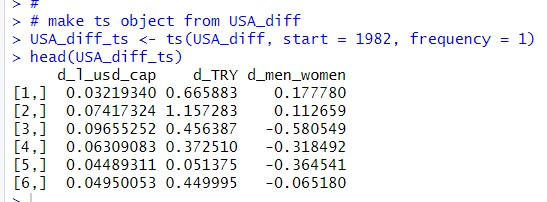Let's use plot() function to make a plot of USA_diff_ts.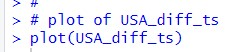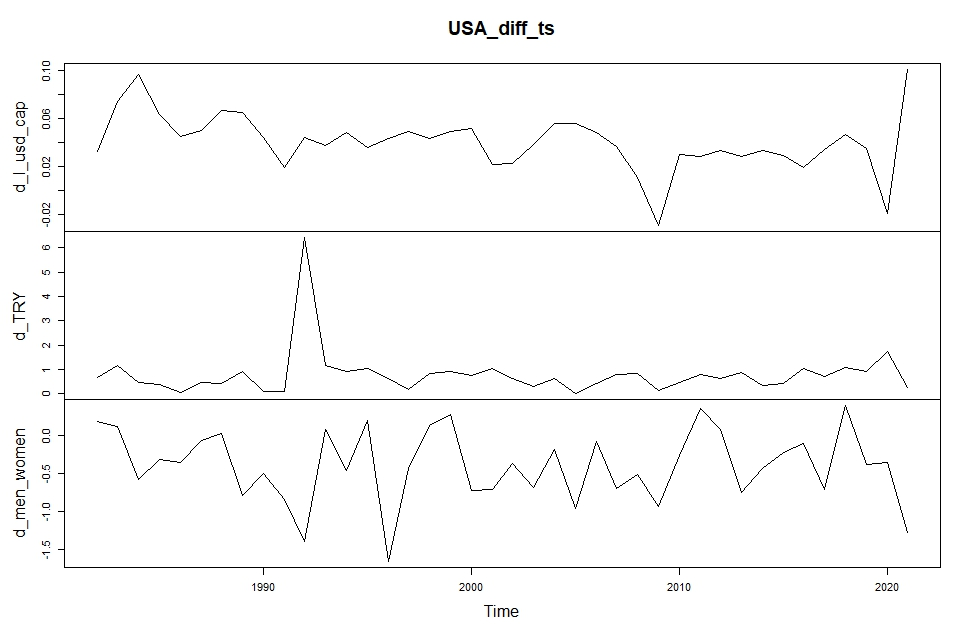Okay, let's make linear regression model.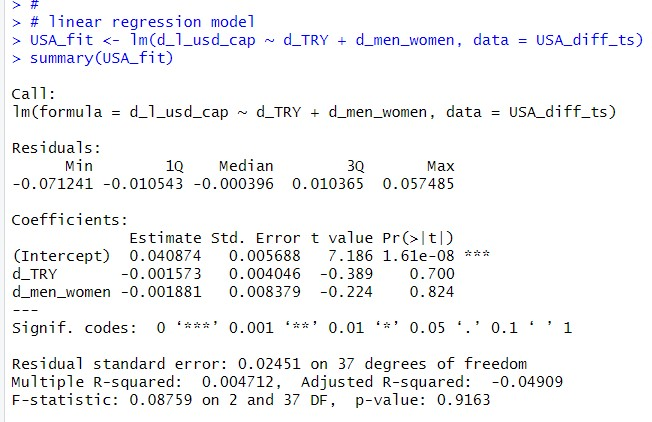The model p-value is 0.9163. So d_TRY and d_men_women are not associated to d_l_usd_cap.

Maybe, lagged variables are associated. Let's check it. I use dynlm package.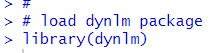I use d() expression in dynlm() function to add lagged variables.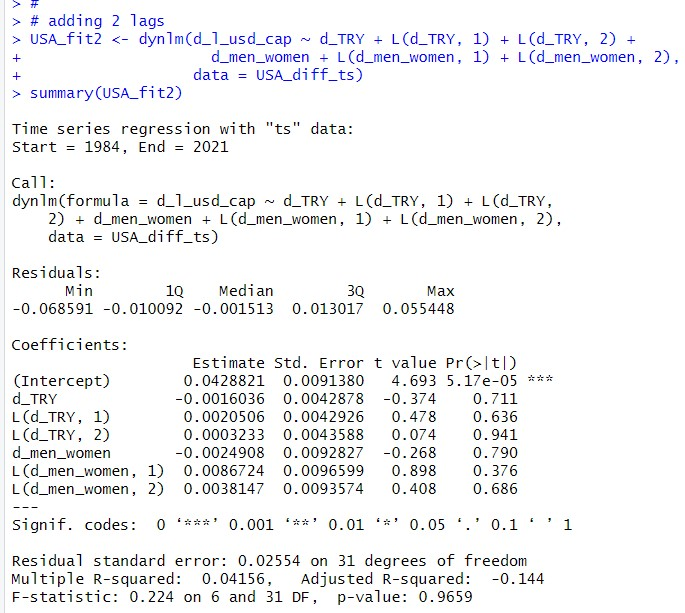This model p-value is 0.9659, so this model is not valid.

I need to think of another model.

That's it. Thank you!

Next post is

To read from the first post,